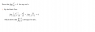# Proving a limit (x^n/(n))

• ecoo

## Homework Statement

Section is on using power series to calculate functions, the problem is on proving the limit, solution is also attached but I do not see how the solution proves the limit.

## Homework Equations

Convergent power series form

## The Attempt at a Solution

I attempted to represent the series with a Maclaurin series, but that leads nowhere. Could someone help explain why the solution given works?

#### Attachments

•Untitled.png
3.1 KB · Views: 415
ecoo said:

## Homework Statement

Section is on using power series to calculate functions, the problem is on proving the limit, solution is also attached but I do not see how the solution proves the limit.

## Homework Equations

Convergent power series form

## The Attempt at a Solution

I attempted to represent the series with a Maclaurin series, but that leads nowhere.
The problem doesn't ask you to find a Maclaurin series -- it just asks you to prove the limit that is given.

ecoo said:
Could someone help explain why the solution given works?
The proof seems very straightforward to me. What part of it do you not understand?

Mark44 said:
The problem doesn't ask you to find a Maclaurin series -- it just asks you to prove the limit that is given.

The proof seems very straightforward to me. What part of it do you not understand?

I don't see how showing that the series converges for all x proves that the limit as n approaches 0 is equal to 0 for all x.

ecoo said:
I don't see how showing that the series converges for all x proves that the limit as n approaches 0 is equal to 0 for all x.
This is true in general for any convergent series. If ##\sum_{n=0}^{\infty}a_n## converges, then ##\lim_{n \to \infty} a_n = 0##. This is sometimes called the n'th term test when stated in its contrapositive form: if the sequence ##a_n## does not converge to zero, then the series ##\sum_{n=0}^{\infty} a_n## diverges.

The converse is false, as shown by the example ##\sum_{n=1}^{\infty}(1/n)##, which diverges even though ##\lim_{n\to\infty}1/n = 0##.

jbunniii said:
This is true in general for any convergent series. If ##\sum_{n=0}^{\infty}a_n## converges, then ##\lim_{n \to \infty} a_n = 0##. This is sometimes called the n'th term test when stated in its contrapositive form: if the sequence ##a_n## does not converge to zero, then the series ##\sum_{n=0}^{\infty} a_n## diverges.

The converse is false, as shown by the example ##\sum_{n=1}^{\infty}(1/n)##, which diverges even though ##\lim_{n\to\infty}1/n = 0##.

That's true, but aren't we trying to prove the limit as n approaches 0, not infinity?

ecoo said:
That's true, but aren't we trying to prove the limit as n approaches 0, not infinity?
What you just wrote makes no sense. They are trying to prove that the limit is zero, as n approaches infinity. More specifically, that ##\lim_{n \to \infty}\frac {x^n}{n!} = 0##. They are using the Ratio Test to prove this.

In addition, this work also proves that the series ##\sum_{n = 0}^{\infty}\frac{x^n}{n!}## is convergent, for the reason jbunniii gave.

Mark44 said:
What you just wrote makes no sense. They are trying to prove that the limit is zero, as n approaches infinity. More specifically, that ##\lim_{n \to \infty}\frac {x^n}{n!} = 0##. They are using the Ratio Test to prove this.

In addition, this work also proves that the series ##\sum_{n = 0}^{\infty}\frac{x^n}{n!}## is convergent, for the reason jbunniii gave.

The first sentence, they are trying to prove the limit is 0 as n approaches 0.

ecoo said:
The first sentence, they are trying to prove the limit is 0 as n approaches 0.
You're right. The type is so small in the image you posted that I misread the 0 for ∞.

I'm pretty sure that the ##n \to 0## under "lim" is a typo. The other work shown suggests strongly that it should be ##\lim_{n \to \infty}## which is what I mistook it to be.

Mark44 said:
You're right. The type is so small in the image you posted that I misread the 0 for ∞.

I'm pretty sure that the ##n \to 0## under "lim" is a typo. The other work shown suggests strongly that it should be ##\lim_{n \to \infty}## which is what I mistook it to be.

Ah, I see. How would one go about prove the limit as n approaches 0, or would it be very hard?

No, it's not hard. If ##x \ne 0##, ##\lim_{x \to 0}\frac {x^n}{n!}## = 1. As ##n \to 0, x^n \to 1##, and 0! is defined to be 1. ##0^0## is indeterminate, though, which is why I qualified what I said about x not being equal to zero.

•ecoo
It doesn't make much sense to talk about the limit as ##n## approaches zero. This is because ##n## is an integer, so the only way it can approach zero is by being zero. So, the limit of a sequence ##a_n## as ##n## approaches zero is simply ##a_0##.

•ecoo
Perhaps try separating the ##|x|## and the ##\frac{1}{n+1}## and you may see why it works for any real x.

It has to do with the value of ##\frac{1}{n+1}## as n approaches infinity.